1.6 Numbers

Numbers are by default denoted in decimal notation. Real (or decimal) numbers are written using engineering or scientific notation (e.g. 0.314E1).

For integer type constants, Free Pascal supports 4 formats:

1. Normal, decimal format (base 10). This is the standard format.
2. Hexadecimal format (base 16), in the same way as Turbo Pascal does. To specify a constant value in hexadecimal format, prepend it with a dollar sign (\$). Thus, the hexadecimal \$FF equals 255 decimal. Note that case is insignificant when using hexadecimal constants.
3. As of version 1.0.7, Octal format (base 8) is also supported. To specify a constant in octal format, prepend it with an ampersand (&). For instance 15 is specified in octal notation as &17.
4. Binary notation (base 2). A binary number can be specified by preceding it with a percent sign (%). Thus, 255 can be specified in binary notation as %11111111.

The following diagrams show the syntax for numbers.

_________________________________________________________________________________________________________
Numbers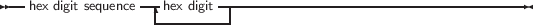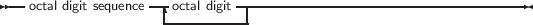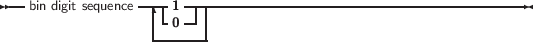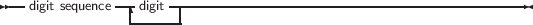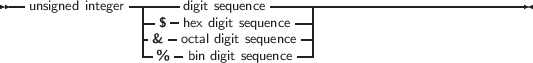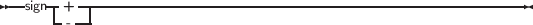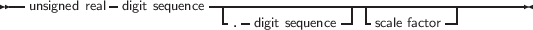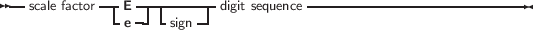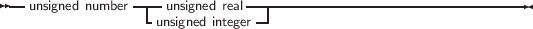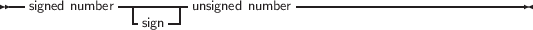___________________________________________________________________

Remark: Octal and Binary notation are not supported in TP or Delphi compatibility mode.